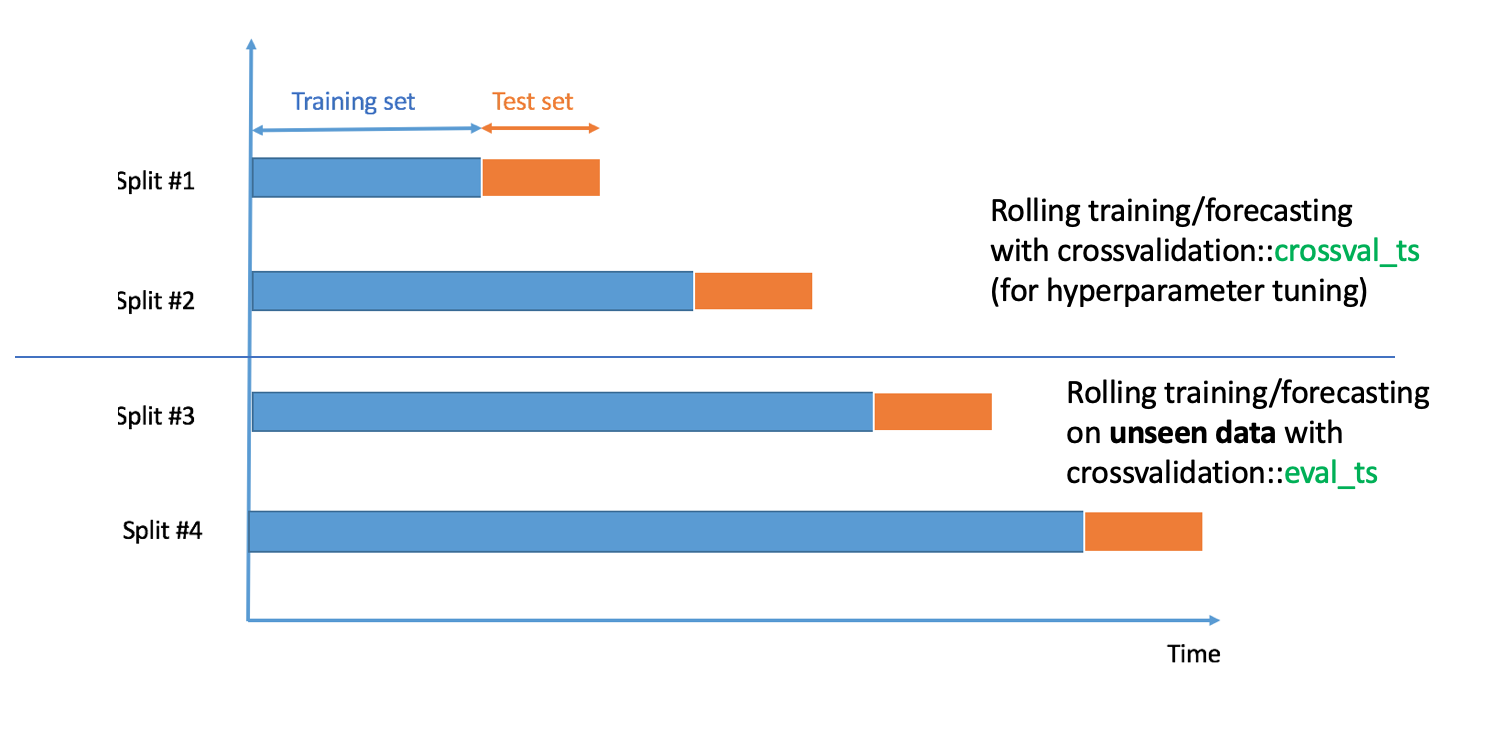In a previous blog post, I presented time series cross-validation with crossvalidation::crossval_ts. The most recent version of package crossvalidation, v0.4.1, contains a new function called eval_ts. How does crossvalidation::eval_ts work? As described in the graph below:crossvalidation::crossval_ts and crossvalidation::eval_ts have the same arguments, except, an argument p for crossval_ts and an argument q for eval_ts. p is the percentage of original data used for cross-validation and hyperparameter tuning, and q is the percentage of unseen data used for model validation. In addition, and most importantly, we must have p + q = 1, to avoid overlapping train/test/validation sets.

Here’s a concrete example of use of crossvalidation::eval_ts :

# Installing packages

options(repos = c(
techtonique = 'https://techtonique.r-universe.dev',
CRAN = 'https://cloud.r-project.org'))

install.packages("crossvalidation")
install.packages("e1071")


library(ahead) # forecasting tool
library(crossvalidation) # cross-validation and evaluation
library(datasets) # dataset Nile
library(e1071) # for svm


# Input data

y <- AirPassengers


# Forecasting function

fcast_func <- function(y, h = 5, kernel=c("linear", "radial"))
{
kernel <- match.arg(kernel)
fit_func = e1071::svm,
fit_params = list(kernel = kernel),
predict_func = predict)
}


We’ll consider that the hyperparameter is kernel, which is either “linear” or “radial”.

# Error metric (Root Mean Squared Error, RMSE)

eval_metric <- function(predicted, observed)
{
return(sqrt(mean((observed - predicted) ^ 2, na.rm = FALSE)))
}


# Cross-validation on 80% of the data

With kernel = "radial"

res_cv_radial <- crossvalidation::crossval_ts(y = y,
fcast_func = fcast_func,
initial_window = 20,
horizon = 10, p=0.8,
eval_metric = eval_metric,
show_progress = FALSE)

 47.29141


With kernel = "linear"

res_cv_linear <- crossvalidation::crossval_ts(y = y,
fcast_func = fcast_func,
fit_params = list(kernel = "linear"),
initial_window = 20,
horizon = 10, p=0.8,
eval_metric = eval_metric,
show_progress = FALSE)
print(median(res_cv_linear))

 44.18955


# Evaluation with the **best hyperparameter, kernel = "linear", on unseen data (q = 1 - p) **

res_eval_linear <- crossvalidation::eval_ts(y = y,
fcast_func = fcast_func,
fit_params = list(kernel = "linear"),
initial_window = 20,
horizon = 10, q=0.2,
eval_metric = eval_metric,
show_progress = FALSE)
print(median(res_eval_linear))

 86.47903